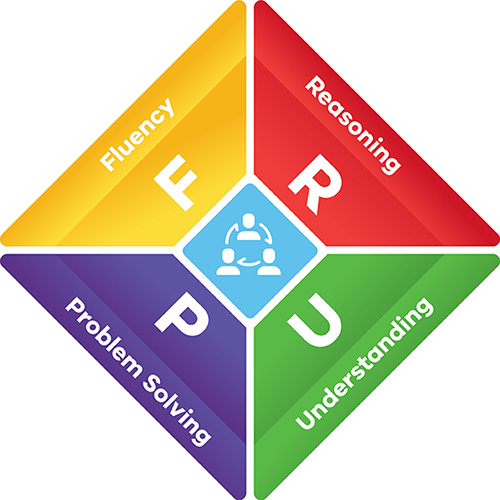### Numeracy Learning

Numeracy Learning at ASPS

Numeracy is the knowledge, skills, behaviours and dispositions that students need in order to use mathematics in a wide range of situations. Children begin to experience and explore mathematical concepts from birth. With support, they participate in mathematical thinking and use mathematical concepts to organise, record and communicate ideas about the world around them.

At Ardeer South Primary School we have daily focused numeracy sessions where a wide range of educational approaches, resources and strategies are used to meet the needs of each child. Teachers use a range of formal and informal assessment and evaluation strategies to measure student progress, learning styles and future needs.

At Ardeer South Primary School we provide an engaging Numeracy Program by:

• Developing a high level understanding of basic ideas and competencies from the Victorian Curriculum in Number & Algebra, Measurement & Geometry and Statistics & Probability
• Embedding the proficiencies (understanding, fluency, problem solving and reasoning) across each strand.
• Developing the skills to quantify, estimate, classify, order, measure, represent, symbolise and problem solve in everyday life.
• Preparing children for a lifetime of mathematical learning and to apply skills to real life situations.
• Providing a supportive learning environment that focuses on skill development and a growth mindset.
• Ensuring that the Numeracy programs of the school meet the learning needs of students at different stages in their development.

### Numeracy focus areas

Children are guided to discover numeracy through six numeracy focus areas, developed from the Victorian numeracy learning progressions and numeracy research:

• developing number sense - quantifying numbers, using additive and multiplicative strategies
• exploring patterns and relationships - using number patterns and thinking algebraically
• using proportional reasoning - operating and interpreting decimals, fractions, percentages, ratios and rates
• understanding and using geometric properties and spatial reasoning
• understanding and using measurement
• exploring chance and data.

The Birth to Level 10 Numeracy Guide is a multi-modal, interactive online guide that includes sections which together form a holistic understanding of numeracy, its relationship with mathematics, what numeracy progression looks like and effective numeracy teaching strategies.

The numeracy guide presents dynamic approaches to developing student numeracy across the learning stages. The guide is used as a roadmap for our teachers as well as a whole-school approach to numeracy learning and development.

### ProficienciesThe Victorian Curriculum: Mathematics identifies a mutual relationship between learning in mathematics and numeracy. This relationship is shown in learning environments through the proficiencies of understanding, fluency, problem solving and reasoning. The proficiencies are fundamental to learning mathematics and working mathematically.

#### Understanding

Building a robust knowledge of mathematical concepts and structures. Students make connections between related concepts and learn to develop new ideas. They develop an understanding of the relationship between the ‘why’ and the ‘how’ of mathematics.

#### Fluency

The development of skills in choosing appropriate procedures, accurately carrying out procedures and using factual knowledge and concepts.

#### Problem solving

The ability of students to make choices, interpret, formulate, model and investigate problem situations, select and use technological functions and communicate solutions.

#### Reasoning

Developing the ability for logical, statistical and probabilistic thinking and actions, such as analysing, proving, evaluating and explaining.

The proficiencies are applied across:

• Number and Algebra
• Measurement and Geometry
• Statistics and Probability.

### High impact teaching strategies (HITS) – numeracy

Ten evidence-based instructional practices that increase student learning.

These resources offer examples of how to use the HITS with a focus on developing students' numeracy and presents examples of HITS best practice from birth to Level 10. The Numeracy HITS have the strongest impact on children and young people's learning when embedded into an ongoing improvement cycle.

At Ardeer South we are committed to embedding these ongoing cycles of improvement to support all numeracy learners to develop skills along a continuum of learning.

NUMERACY AT HOME

Find information, advice and activities for families to help their child’s numeracy learning at home.

http://numeracyguidedet.global2.vic.edu.au/numeracy-at-home/